# AQA A Level Maths: Statistics复习笔记4.1.1 Discrete Probability Distributions

### Discrete Random Variables

#### What is a discrete random variable?

• A random variable is a variable whose value depends on the outcome of a random event
• The value of the random variable is not known until the event is carried out (this is what is meant by 'random' in this case)
• Random variables are denoted using upper case letters (X , Y , etc )
• Particular outcomes of the event are denoted using lower case letters ( x, y, etc)
•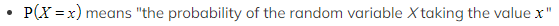A discrete random variable (often abbreviated to DRV) can only take certain values within a set
• Discrete random variables usually count something
• Discrete random variables usually can only take a finite number of values but it is possible that it can take an infinite number of values (see the examples below)
• Examples of discrete random variables include:
• The number of times a coin lands on heads when flipped 20 times
(this has a finite number of outcomes: 0,1,2,…,20)
• The number of emails a manager receives within an hour
(this has an infinite number of outcomes: 1,2,3,…)
• The number of times a dice is rolled until it lands on a 6
(this has an infinite number of outcomes: 1,2,3,…)
• The number on a bingo ball when one is drawn at random
(this has a finite number of outcomes: 1,2,3…,90)

### Probability Distributions (Discrete)

#### What is a probability distribution?

• A discrete probability distribution fully describes all the values that a discrete random variable can take along with their associated probabilities
• This can be given in a table (similar to GCSE)
• Or it can be given as a function (called a probability mass function)
•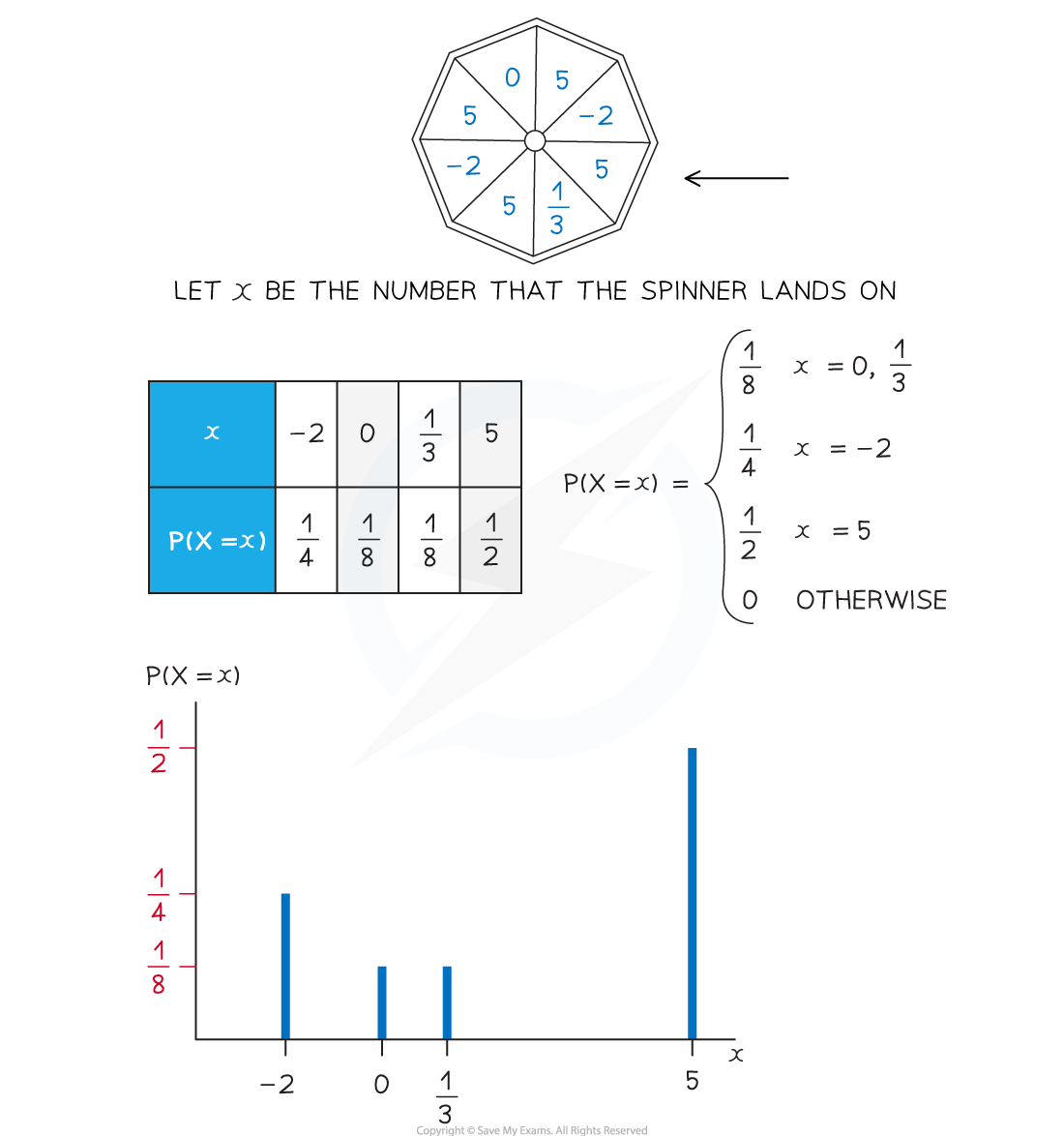### Cumulative Probabilities (Discrete)

#### How do I calculate probabilities using a discrete probability distribution?

• First draw a table to represent the probability distribution
• If it is given as a function then find each probability
• If any probabilities are unknown then use algebra to represent them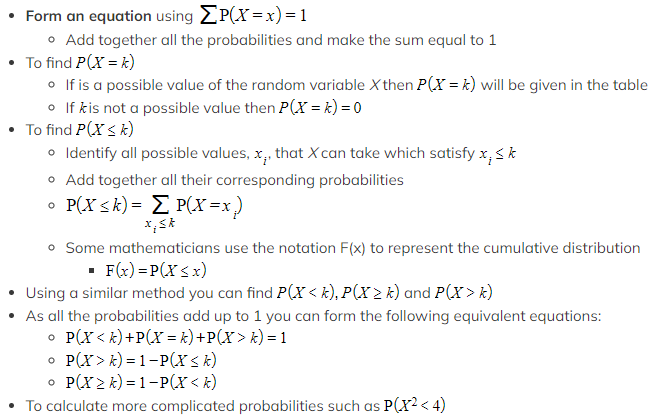Identify which values of the random variable satisfy the inequality or event in the brackets
• Add together the corresponding probabilities

#### Worked Example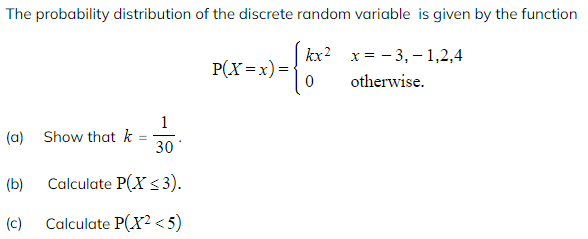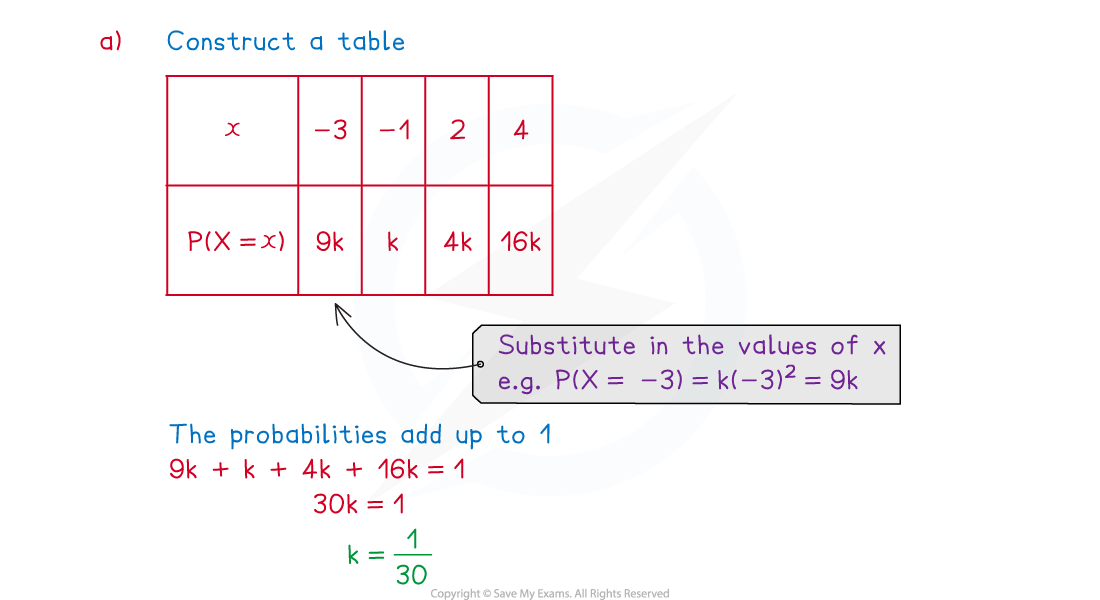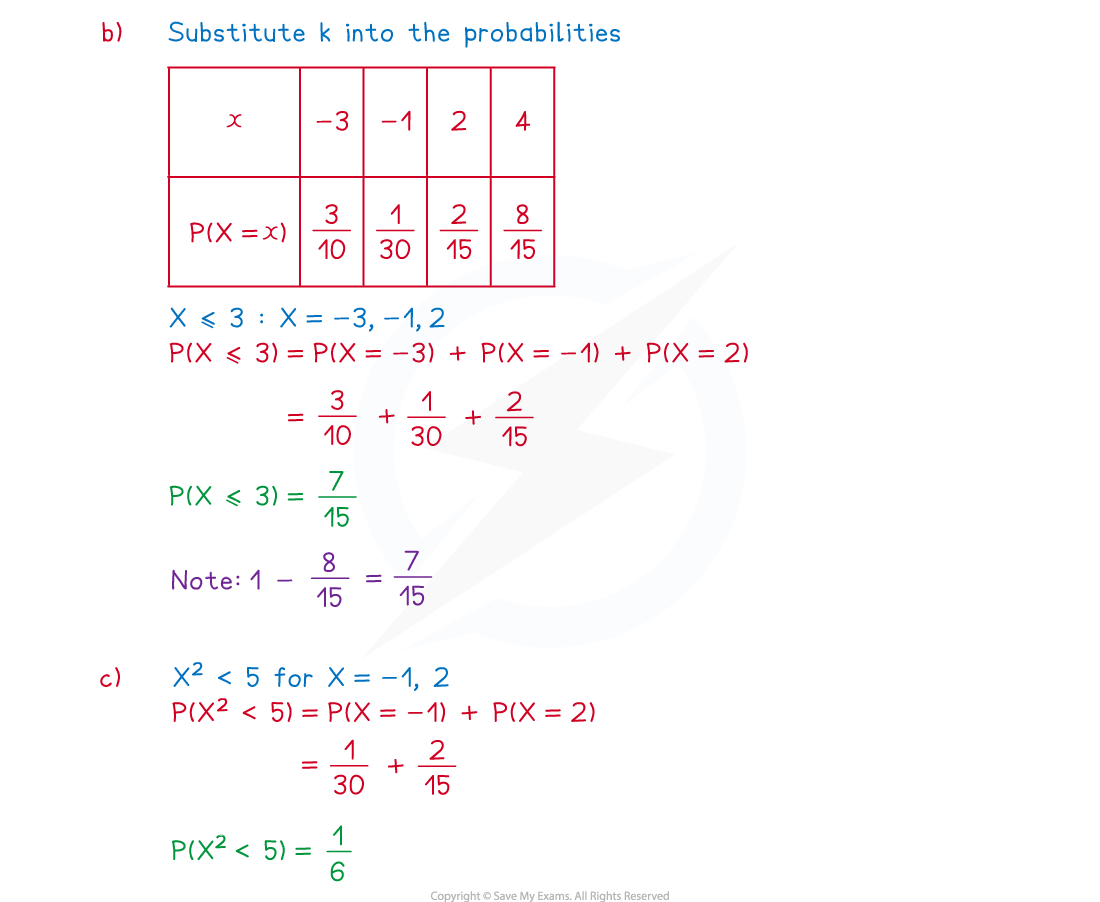#### Exam Tip

• Try to draw a table if there are a finite number of values that the discrete random variable can take
• When finding a probability, it will sometimes be quicker to subtract the probabilities of the unwanted values from 1 rather than adding together the probabilities of the wanted values
• Always make sure that the probabilities are between 0 and 1, and that they add up to 1!